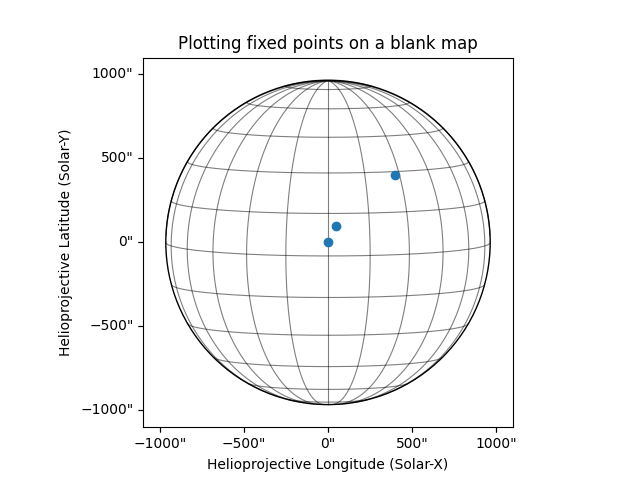# Plot positions on a blank map#

This example showcases how to plot positions on a blank map. It is often useful to plot coordinate positions of events on a blank helioprojective coordinate map. In this example, we create an empty map with a WCS defined by a helioprojective frame as observed from Earth at a certain time, and show how you can plot different coordinates on it.

import matplotlib.pyplot as plt
import numpy as np

import astropy.units as u
from astropy.coordinates import SkyCoord

import sunpy.map
from sunpy.coordinates import frames


First we will create a blank map using with an array of zeros. Since there is no WCS information, we will need to construct a header to pass to Map.

data = np.full((10, 10), np.nan)

skycoord = SkyCoord(0*u.arcsec, 0*u.arcsec, obstime='2013-10-28',
observer='earth', frame=frames.Helioprojective)

# Scale set to the following for solar limb to be in the field of view

# Use sunpy.map.Map to create the blank map


Now we have constructed the map, we can plot it and mark important locations to it. Initialize the plot and add the map to it

fig = plt.figure()
blank_map.plot(axes=ax)
blank_map.draw_limb(axes=ax, color="k")
blank_map.draw_grid(axes=ax, color="k")

<CoordinatesMap with 2 world coordinates:

index aliases    type   unit    wrap   format_unit visible
----- ------- --------- ---- --------- ----------- -------
0     lon longitude  deg 180.0 deg         deg     yes
1     lat  latitude  deg      None         deg     yes

>


Coordinates that are being plotted - (0, 0), (50, 100) and (400, 400).

xc = [0, 50, 400] * u.arcsec
yc = [0, 100, 400] * u.arcsec


Place and mark coordinates on the plot.

coords = SkyCoord(xc, yc, frame=blank_map.coordinate_frame)
p = ax.plot_coord(coords, 'o')
# Set title.
ax.set_title('Plotting fixed points on a blank map')

plt.show()Total running time of the script: (0 minutes 0.559 seconds)

Gallery generated by Sphinx-Gallery